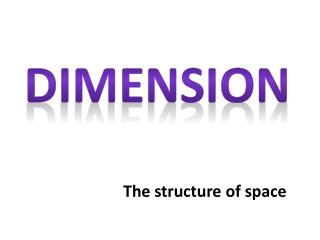DownloadDownload PresentationDimension

# Dimension

Download Presentation## Dimension

- - - - - - - - - - - - - - - - - - - - - - - - - - - E N D - - - - - - - - - - - - - - - - - - - - - - - - - - -
##### Presentation Transcript

1. Dimension The structure of space

2. Dimension Each dimension is perpendicular to the other Length Width Height Time

3. Cube Hypercube Tesseract

4. Spacetime 4th Dimension

5. Net of cube

6. Unfolded net of hypercube or tesseract

7. Model of Hypercube 28 cardboard squares. Tape them, four at a time To make seven partial cubes. Partial cubes have no tops or bottoms, they're like square tubes. Tape the seven partial cubes together as shown

8. 2-Sphere Projection of 3D

9. Latitude

10. Hyperspheres of dimension n > 2 0-sphere is the pair of points at the ends of a (one-dimensional) line segment 1-sphere is the circle, which is the one-dimensional circumference of a (two-dimensional) disk in the plane, 2-sphere is the two-dimensional surface of a (three-dimensional) ball in three-dimensional space.

11. String Theory

12. Sliceform of Sphere

13. Equator in yellow

14. Add equator and latitudes Shown in yellow